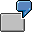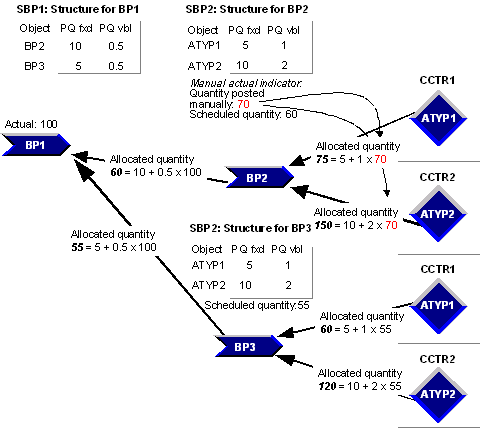Example Template-Allocation Process (Actual)Receiver business process BP1 is stored with sender processes BP2 and BP3 in template SBP1. Both sender processes are processes based on template SBP2. This template stores activity input ATYP1 from cost center CCTR1 and activity input ATYP2 from cost center CCTR2.

Receiver BP1 has a process quantity of 100 posted in actual. Sender BP2 uses a fixed actual process quantity of 10 with an actual quantity factor of 0.5. Sender BP3 uses a fixed actual process quantity of 5 with an actual quantity factor of 0.5.

Sender BP2 is stored in the process master data with an active Actual quantity indicator and a manual posting of 70.

In template SBP2, ATYP1 uses a fixed actual process quantity of 5 with an actual quantity factor of 1. ATYP2 uses a fixed actual process quantity of 5 with an actual quantity factor of 2.Actual Allocation of Process Quantities

Allocation of process quantities results in the calculation and allocation of the following: Sender BP2: Scheduled actual quantity 60 = Fixed period quantity 10 + Variable period quantity 50 (0.5 X 100) Sender BP3: Scheduled actual quantity 55. = Fixed period quantity 5 + Variable period quantity 50 (0.5 X 100)

Further allocation calculations result in senders BP2 and BP3 becoming receivers as well. Allocation to receivers BP2 and BP3 uses different process quantities.

Because BP2 has an active Actual quantity indicator, further allocations use the manual posting of 70 for the actual process quantity: BP3 receives the process quantity of 55 scheduled on receiver process BP1.

The allocation of process quantities shows that the actual quantity for ATYP 1 on Cctr 1 scheduled by receiver process BP2 equals 75. ATYP1: Scheduled actual quantity 60 = Fixed period quantity 5 + Variable period quantity 70 (1 X 70) The actual quantity scheduled through the receiver pocess BP2 from activity type AT2 and cost center CCTR 2 is 150. ATYP1: Scheduled actual quantity 60 = Fixed period quantity 10 + Variable period quantity 140 (2 X 70)

BP3 uses the scheduled process quantity 55 from receiver BP1: ATYP1: Scheduled actual quantity 60 = Fixed period quantity 5 + Variable period quantity 55 (1 X 55) The actual quantity scheduled through the receiver process BP3 from activity type AT2 and cost center CCTR 2 is 120. ATYP1: Scheduled actual quantity 60 = Fixed period quantity 10 + Variable period quantity 110 (2 X 55)